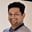Related Tags

java

# How to calculate the area of a triangle with three pointsTarun Telang

## Overview

We can use the Java program to calculate the area of a triangle, given that we have the coordinates of the three vertices as input.

We can use Heron's formula to calculate the area of a triangle.

The formula for the area is as follows:

where (x1, y1), (x2, y2), and (x3, y3) are the coordinates of the vertices.

### Example

For example, let's say we have a triangle with vertices at (0, 0), (2, 5), and (5, 2). This would be represented in X-Y coordinates as in the figure below:

Triangle with vertices at P1(0,0), P2(2, 5), P3(5,2)

We can plug these values into the formula to calculate the area:

### Code

We can use the below Java code to calculate the area:

class AreaOfTriangle {

// A function to calculate the area of triangle
// formed by (x1, y1), (x2, y2) and (x3, y3) coordinates
static double area(int x1, int y1, int x2, int y2,
int x3, int y3)
{
return Math.abs((x1 * (y2 - y3) + x2 * (y3 - y1) +
x3 * (y1 - y2)) / 2.0);
}

// Driver Code
public static void main(String[] args)
{
int x1 = 0, y1 = 0;
int x2 = 2, y2 = 5;
int x3 = 5, y3 = 2;

double area = area(x1, y1, x2, y2, x3, y3);
System.out.println("Area of Triangle is " + area);
}
}
A Java program to calculate the area of the triangle, given three points as input

### Explanation

In the above code, we'll use Heron's formula to calculate the area of a triangle given three points.

The function area takes six parameters, (x1, y1, x2, y2, x3, y3), which are the coordinates of the three points, and returns the value of the area.

We also use the Math.abs() function to take care of negative values that might be returned by the function. The main() method calls the area() function with six arguments, which are the x and y coordinates of the three points. The value returned by area is stored in a variable named area and is printed on the screen.

Output: Area of triangle is 10.5

Time complexity: O(1)

Space complexity: O(1)

RELATED TAGS

java

CONTRIBUTORTarun Telang
RELATED COURSES

View all Courses

Keep Exploring

Learn in-demand tech skills in half the time